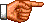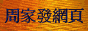# 點算的奧秘：非齊次遞歸關係的解

《點算的奧秘：齊次遞歸關係的解》中，筆者介紹了求解「常系數一元線性齊次遞歸關係」的方法，在本網頁筆者將介紹求解「常系數一元線性非齊次遞歸關係」的方法。在這裡首先重溫「非齊次遞歸關係」的定義。當我們把一個「遞歸關係」寫成標準形式，即把含有an、an−1 ...等的項統統移到等號左邊，並把其餘的項移到等號右邊後，如果在等號右邊的項不等於0，則該「遞歸關係」是「非齊次」的。我們把這個在等號右邊的非零項稱為「非齊次遞歸關係」的「非齊次部分」。

「未定系數法」乃基於以下假設：「非齊次遞歸關係」的「特解」與該「遞歸關係」的「非齊次部分」具有相同的形式，兩者僅在系數方面有差異。因此，假如給定「遞歸關係」的「非齊次部分」是「指數函數」3 × 2n，我們便假設pn也具有相同的形式，即pn = A × 2n；假如給定「遞歸關係」的「非齊次部分」是「3階多項式」n3 − 1，我們便假設該「遞歸關係」的「特解」也具有相同的形式，即pn = An3 + Bn2 + Cn + D (註2)。假如給定「遞歸關係」的「非齊次部分」具有較複雜的形式(例如混合了「指數函數」和「多項式」)，則pn亦要具有同類型的複雜形式，現把這些情況總結成下表：

an = 2an−1 + n − 1

an − 2an−1 = n − 1

 An + B = 2[A(n − 1) + B] + n − 1 = 2An − 2A + 2B + n − 1 = (2A + 1)n − 2A + 2B − 1

 A = 2A + 1 B = − 2A + 2B − 1

pn = −n − 1 □

an = gn + pn

a1 = 0

an − 2an−1 = 0

x − 2 = 0

gn = C × 2n

an = C × 2n − n − 1

 a1 = C × 21 − 1 − 1 即，2C − 2 = 0

an = 2n − n − 1

a4 = 24 − 4 − 1 = 11

 an = 2an−1 + 3n−1 − 2n−1 ，若n > 1 a1 = 0

an − 2an−1 = 3n−1 − 2n−1

x − 2 = 0

gn = C × 2n

pn = A × 3n + B × 2n (註3)

pn = A × 3n + Bn × 2n

 A3n + Bn2n = 2[A3n−1 + B(n − 1)2n−1] + 3n−1 − 2n−1 3A(3n−1) + 2Bn(2n−1) = (2A + 1)3n−1 + (−2B − 1)2n−1 + 2Bn(2n−1) 3A(3n−1) = (2A + 1)3n−1 + (−2B − 1)2n−1

 3A = 2A + 1 0 = −2B − 1

 pn = 3n − n / 2 × 2n = 3n − n × 2n−1

an = C × 2n + 3n − n × 2n−1

 a1 = C × 20 + 30 − 0 × 20−1 即，C + 1 = 0

an = −2n + 3n − n × 2n−1

a4 = −24 + 34 − 4 × 24−1 = 33

 an − 4an−1 + 5an−2 − 2an−3 = 2 + 2n，若n > 2 a0 = 6，a1 = 20，a2 = 52

x3 − 4x2 + 5x − 2 = 0

(x − 1)2(x − 2) = 0

 gn = C × 1n + Dn × 1n + E × 2n = C + Dn + E × 2n

pn = A + B × 2n

pn = An2 + Bn × 2n

 An2 + Bn2n − 4[A(n − 1)2 + B(n − 1)2n−1] + 5[A(n − 2)2 + B(n − 2)2n−2] − 2[A(n − 3)2 + B(n − 3)2n−3] = An2 + Bn2n − 4An2 + 8An − 4A − 4Bn2n−1 + 4B2n−1 + 5An2 − 20An + 20A + 5Bn2n−2 − 10B2n−2 − 2An2 + 12An − 18A − 2Bn2n−3 + 6B2n−3 = (1 − 4 + 5 − 2)An2 + (8 − 20 + 12)An + (−4 + 20 − 18)A + (8 − 16 + 10 − 2)Bn2n−3 + (16 − 20 + 6)B2n−3 = −2A + 2B2n−3

−2A + 2B2n−3 = 2 + 8 × 2n−3

 pn = −n2 + 4n × 2n = −n2 + n × 2n+2

an = C + Dn + E × 2n − n2 + n × 2n+2

 a0 = C + D × 0 + E × 20 − 02 + 0 × 20+2 即，C + E = 6

 a1 = C + D × 1 + E × 21 − 12 + 1 × 21+2 即，C + D + 2E + 7 = 20

 a2 = C + D × 2 + E × 22 − 22 + 2 × 22+2 即，C + 2D + 4E + 28 = 52

 an = 2 + 3n + 4 × 2n − n2 + n × 2n+2 = 2 + 3n − n2 + (1 + n) × 2n+2

a3 = 2 + 3 × 3 − 32 + (1 + 3) × 23+2 = 130
a4 = 2 + 3 × 4 − 42 + (1 + 4) × 24+2 = 318

a3 = 4a2 − 5a1 + 2a0 + 2 + 23 = 4 × 52 − 5 × 20 + 2 × 6 + 2 + 23 = 130
a4 = 4a3 − 5a2 + 2a1 + 2 + 24 = 4 × 130 − 5 × 52 + 2 × 20 + 2 + 24 = 318